# Dependency Injection in Javascript

This article explores dependency injection in Javascript using higher-order functions and compares it against dependency injection in classes.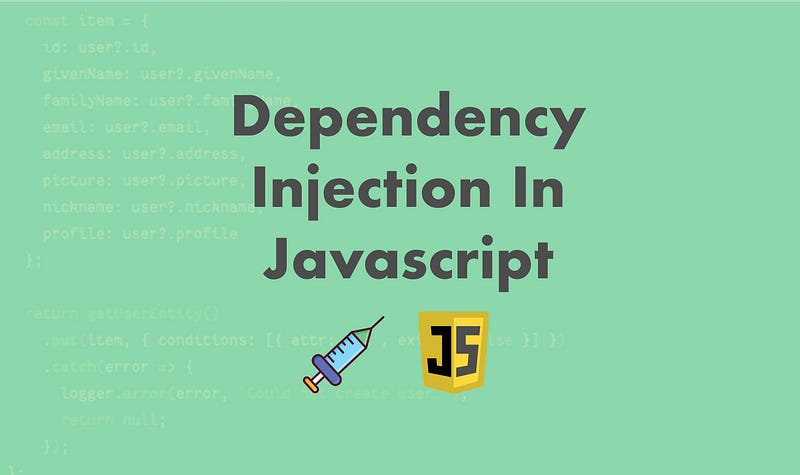Dependency injection (DI) is a programming pattern in which a dependency is passed using the parameters instead of instantiating it within the function or class. DI enables creating isolated individual components within application code and makes it easy to switch those dependencies in the future as the requirement changes. Passing parameters as a dependency also allows to easily unit test those components in isolation by injecting their mocked version.

This article explores dependency injection in Javascript using higher-order functions and compares it against classes. This pattern is appropriate in any language that supports standalone function definitions.

For the rest of the article, let us assume that we are building a simple hypothetical application with an `Course` entity and the Course has `Lesson`. `AWS DynamoDB` stores the Course and `S3` bucket stores lessons as JSON objects.

First, to understand this pattern, let us explore how we would achieve dependency injection using classes, then we will explore some examples of this pattern using javascript functions.

### Dependency Injection using classes

First, let us explore dependency injection using classes, then we can compare it with dependency injection using higher-order functions.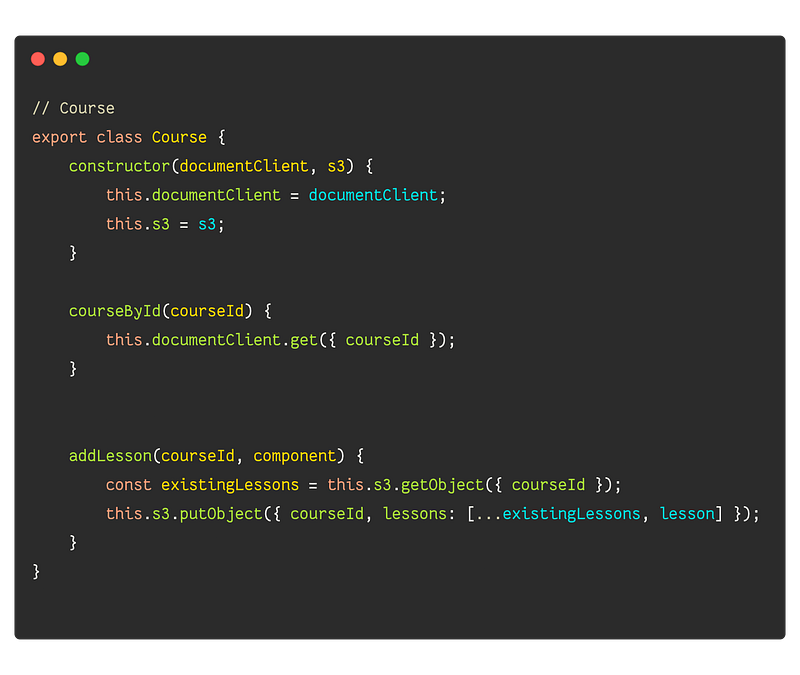DI using classes

In the above example, we have defined a Course class that has two methods. `courseById` gets the course from DynamoDB and `addLesson` adds a given lesson to S3. We have also injected `documentClient` and `S3` from `aws-sdk`.

Now that we have this class with some methods, let us see how we could use it. To use this in our application, we first need to create an instance of the `Course`. Unless we are using a dependency injection framework, typically, we achieve this using a `Factory` class.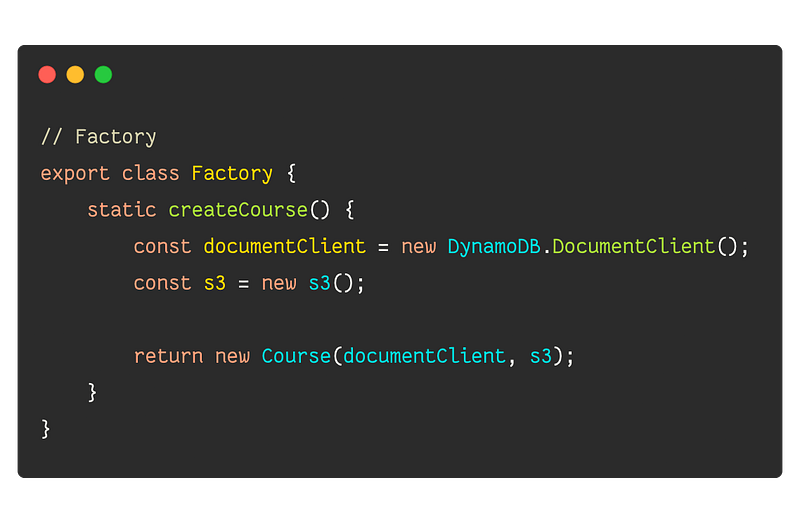We can then use the `Factory.createCourse` in our application to create an instance, of course, then invoke the appropriate method. We could extend `Factory` to include other static methods to create an instance of other entities like `Lesson`.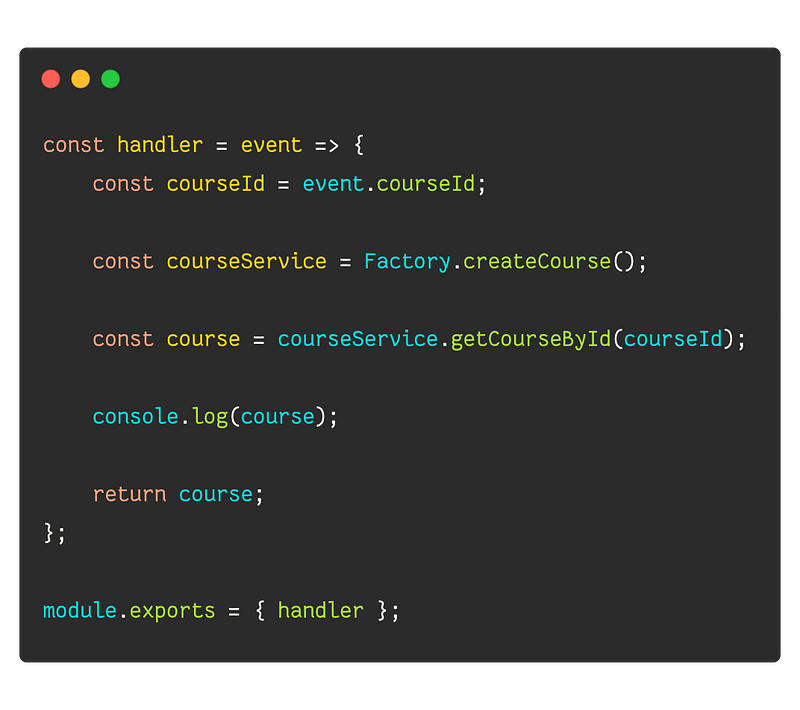Now, if the requirement changes in the future and we need to store `Course` in some other store, then we can update the `Factory` or `Course` Moreover, our business logic will be untouched.

### Dependency Injection using the higher-order function

With the examples using classes, notice that we would always need to have an instance of `S3` and `DocumentClient` before we can have an instance of `Course`.

Imagine a scenario where we have a webhook or `AWS Lambda Function` whose job is to `getCourseById`. In this scenario, we will end up creating an instance `S3` even though we will not use it in this request.

In an actual application, there might be much more complex logic to setup and teardown each dependency which might degrade the performance.

Let us explore dependency injection at the function level.

The premise of this pattern is based on a function that returns another function ( higher-order function). The higher-order function accepts all the dependencies that are required for the child function to perform its job.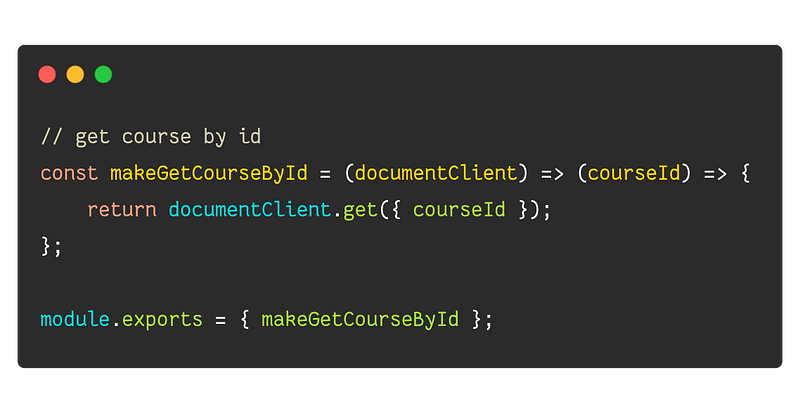DI with a higher-order function

In the above example, we create a function called `makeGetCourseById`. Its job is to create a function that we can use to get a course by id.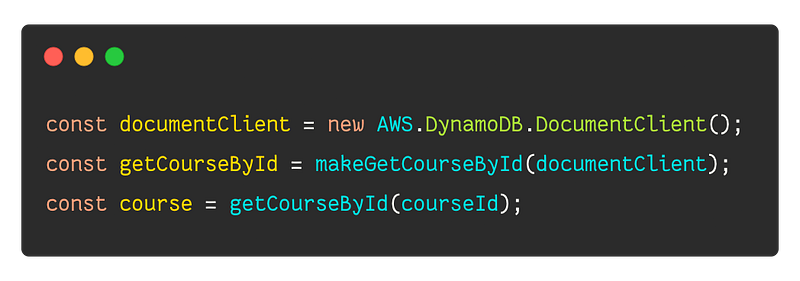We can then use the higher-order function as above in our application code. We can follow the same pattern for `addLesson`.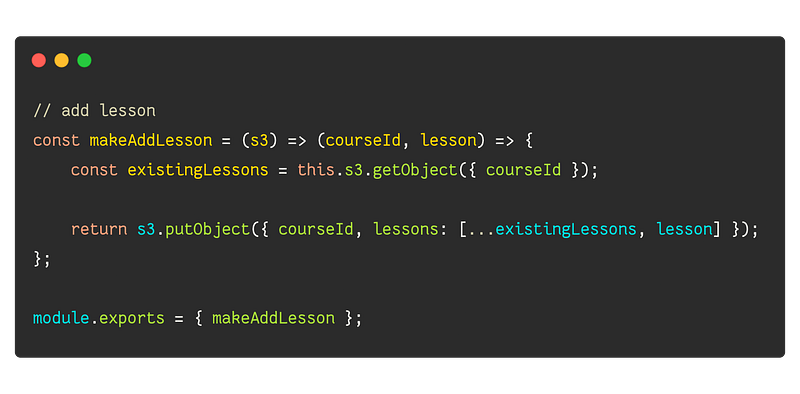DI with a higher-order function

The idea with the higher-order function is that we create individual functions like `getCourseById` or `addLesson` once and pass the instance around in our application. If we did not use the higher-order components, then we would need to pass an instance of `documentClient` or `s3` every time we want to get course by id.

The following code sample demonstrates how we can use the higher-order function in `AWS Lambda Handler`.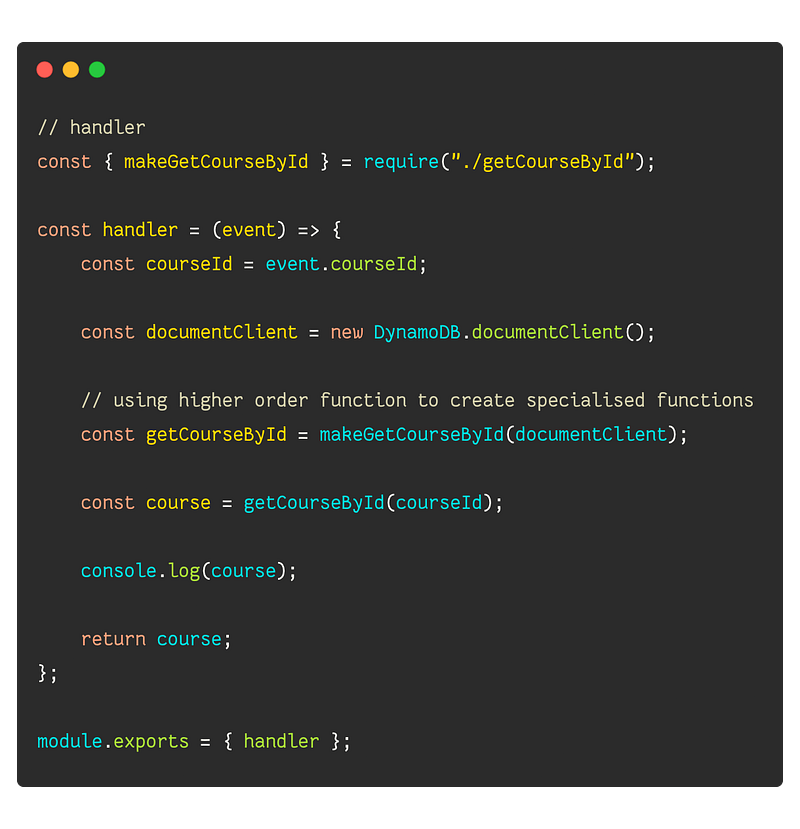### Creating services with higher-order functions

There will be scenarios where we might need to create a collection of methods that we can use without initializing each method. For example, we want to attach a service with a collection of methods in the `GraphQL` context to use them in GraphQL resolvers.

To accomplish this, we can create a function that returns an object with a collection of functions.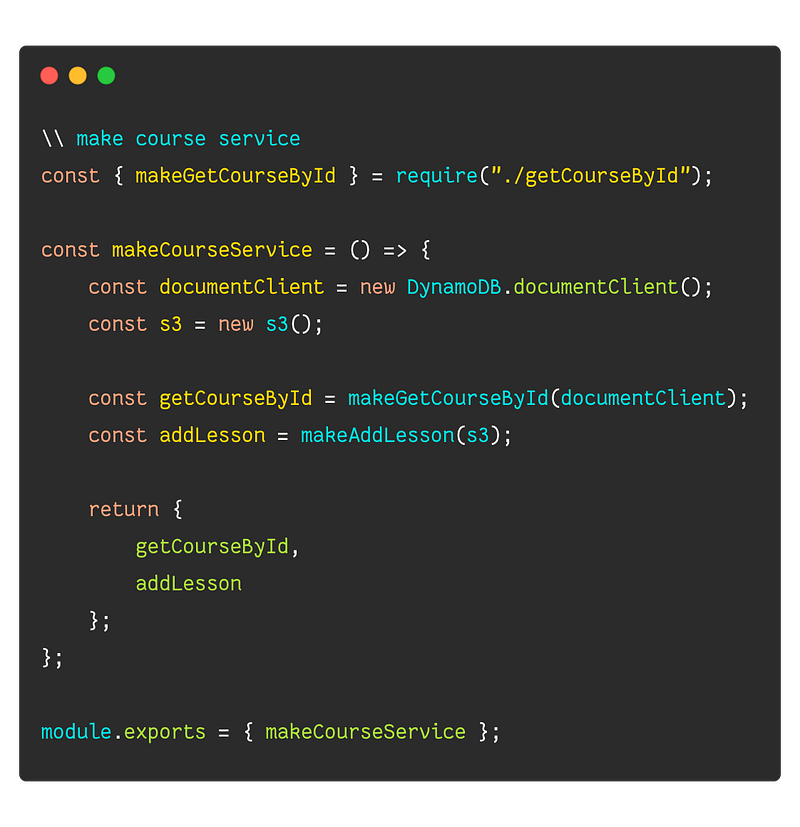We can then use it in our application code as following. Keep in mind that we create an instance of `courseService` once per application execution if possible or can even cache it if necessary.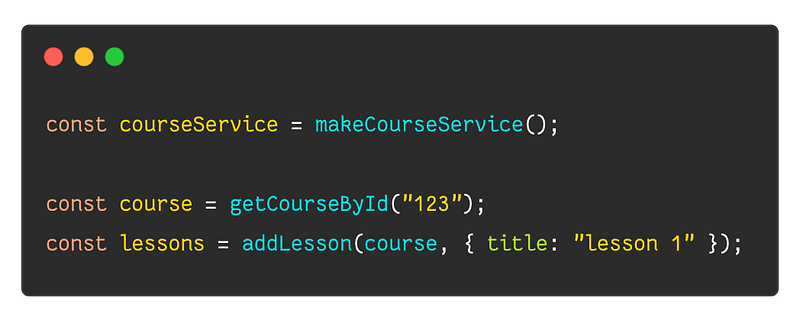### Passing methods around

Often we will run into scenarios where we might want to use the higher-order function inside another higher-order function. For example, we might want to check if the Course exists before adding a lesson. We can approach this by accepting `getCourseById` and `S3` in the parameters.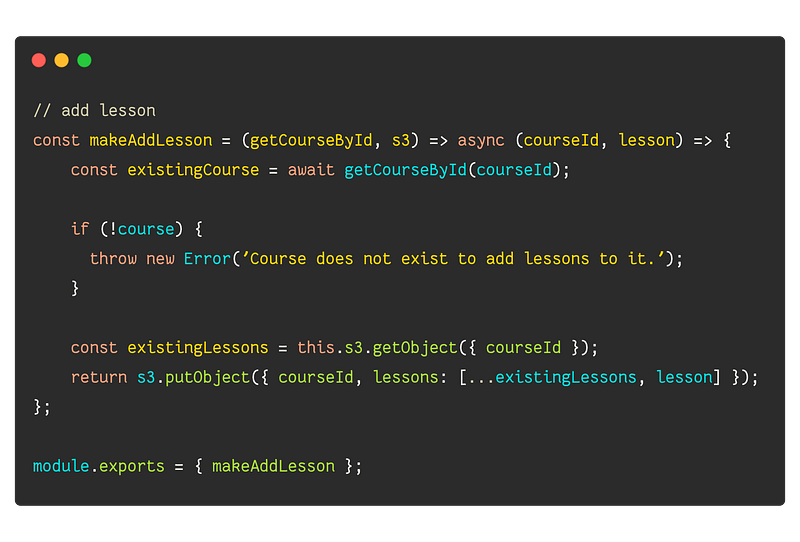The application code will look like the following: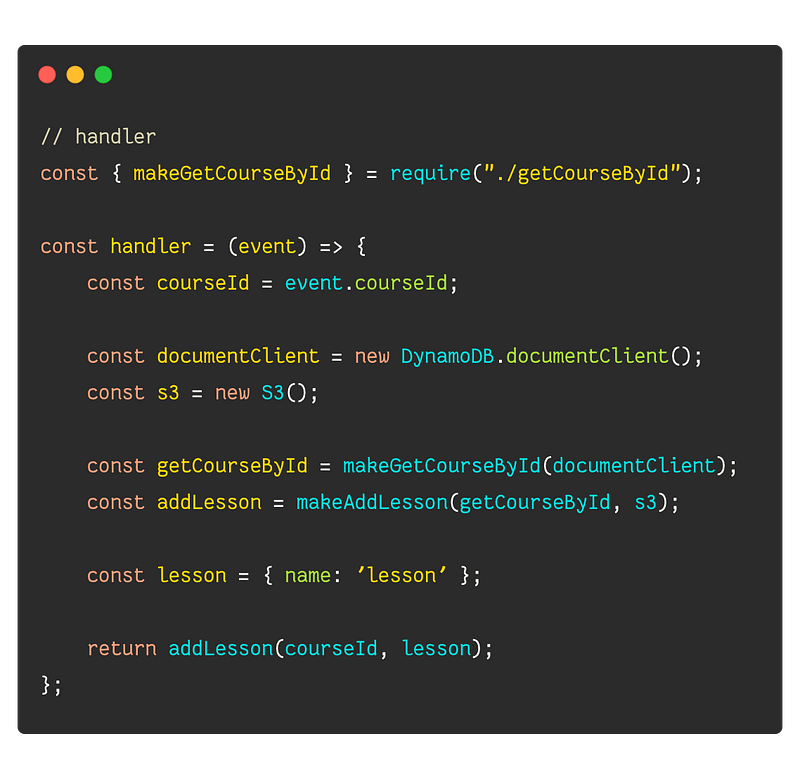### Summary

We explored two different ways to achieve dependency injection in Javascript. One way of achieving dependency injection in Javascript is to use `Class` and a `Factory`. The other approach is to use higher-order functions to achieve granular injection on the function level. Depending on the application use cases, it is best to pick one approach and stick to it. Higher-order functions are relatively simple to work with and are more flexible than Javascript classes.